International
Tables for
Crystallography
Volume C
Mathematical, physical and chemical tables
Edited by E. Prince

International Tables for Crystallography (2006). Vol. C, ch. 9.8, pp. 942-943

## Section 9.8.5.2. The incommensurate versus the commensurate case

T. Janssen,a A. Janner,a A. Looijenga-Vosb and P. M. de Wolffc

aInstitute for Theoretical Physics, University of Nijmegen, Toernooiveld, NL-6525 ED Nijmegen, The Netherlands,bRoland Holstlaan 908, NL-2624 JK Delft, The Netherlands, and cMeermanstraat 126, 2614 AM, Delft, The Netherlands

#### 9.8.5.2. The incommensurate versus the commensurate case

| top | pdf |

As said earlier, it sometimes makes sense also to use the description as developed for incommensurate crystal phases for a (commensurate) superstructure. In fact, very often the modulation wavevector also shows, in addition to continuously varying (incommensurate) values, several rational values at various phase transitions of a given crystal or in different compounds of a given structural family. In these cases, there is three-dimensional space-group symmetry. Generally, the space groups of the various phases are different. The description as used for incommensurate phases then gives the possibility of a more unified characterization for the symmetry of related modulated crystal phases. In fact, the theory of higher-dimensional space groups for modulated structures is largely independent of the assumption of irrationality. Only some of the statements need to be adapted. The most important change is that there is no longer a one-to-one correspondence between the points of the reciprocal lattice* and its projection on V defining the positions of the Bragg peaks. Furthermore, the projection of the latticeon the space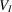forms a discrete set. The latter means the following. For an incommensurate modulation, the incommensurate structure, which is the intersection of a periodic structure with the hyperplane, is also the intersection of the same periodic structure with a hyperplane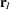= constant , where this constant is of the form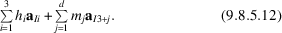Because for an incommensurate structure these vectors form a dense set in, the phase of the modulation function with respect to the basic structure is not determined. For a commensurate modulation, however, the points (9.8.5.12)form a discrete set, even belong to a lattice, and the phase (or the phases) of the modulation are determined within vectors of this lattice. Notice that the grid of this lattice becomes finer as the denominators in the rational components become larger.

Whenis a (3 + d)-dimensional superspace group, its elements, in general, do not leave the ordinary space V invariant. The subgroup of all elements that do leave V invariant, when restricted to V, is a group of distance-preserving transformations in three dimensions and thus a subgroup of E(3), the three-dimensional Euclidean group. In general, this subgroup is not a three-dimensional space group. It is so when the modulation wavevectors all have rational components only, i.e. when σ is a matrix with rational entries. Because the phase of the modulation function is now determined (within a given rational number smaller than 1), the space group depends in general on this phase.

As an example, consider a one-dimensional modulation of a basic structure with orthorhombic space group Pcmn. Suppose that the modulation wavevector is γc*. Then the mirror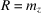perpendicular to the c axis is combined with== −1. Suppose, furthermore, that the glide reflection perpendicular to the a axis and the b mirror are both combined with a phase shift. In terms of the coordinates x, y, z with respect to the a, b and c axes, and internal coordinate t, the generators of the (3 + 1)-dimensional superspace group Pcmn(00γ)ss0 act as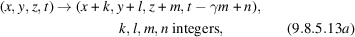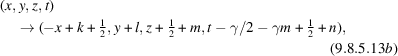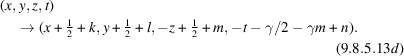Note that these positions are referred to a split basis (i.e. of basis vectors lying either in V or in) and not to a basis of the lattice. When the superstructure is the intersection of a periodic structure with the plane at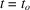, its three-dimensional space group follows from equation (9.8.5.13)by the requirement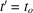. When γ has the rational value r/s with r and s relatively prime, the conditions for each of the generators to give an element of the three-dimensional space group are, respectively: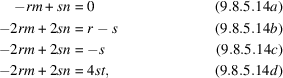for m, n, r, s integers and t real. These conditions are never satisfied simultaneously. It depends on the parity of both r and s which element occurs, and for the elements with= −1 it also depends on the value of the `phase' t, or more precisely on the product τ = 4st. The translation group is determined by the first condition as in (9.8.5.14a). Its generators are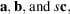where the last vector is the external part of the lattice vector s(c, −r/s) + r(0, 1). The other space-group elements can be derived in the same way. The possible space groups are:

γ = r/sτ even integerτ odd integerotherwise
r even, s odd212121 1121
r odd, s even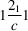21cn 1c1
r odd, s oddc21n c11

In general, the three-dimensional space groups compatible with a given (3 + d)-dimensional superspace group can be determined using analogous equations.

As one can see from the table above, the orthorhombic (3 + d)-dimensional superspace group leads in several cases to monoclinic three-dimensional space groups. The lattice of main reflections, however, still has orthorhombic point-group symmetry. Description in the conventional way by means of three-dimensional groups then neglects some of the structural features present. Even if the orthorhombic symmetry is slightly broken, the orthorhombic basic structure is a better characterization than a monoclinic one. Note that in that case the superspace-group symmetry is also only an approximation.

When the denominators of the wavevector components become small, additional symmetry operations become possible. Because the one-to-one correspondence between* and M* is no longer present, there may occur symmetry elements with trivial action in V but with nontrivial transformation in. For d = 1, these possibilities have been enumerated. The corresponding Bravais classes are given in Table 9.8.3.2(b).